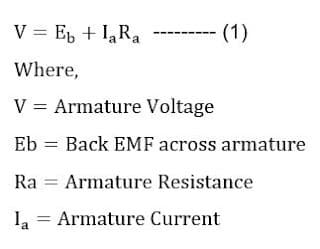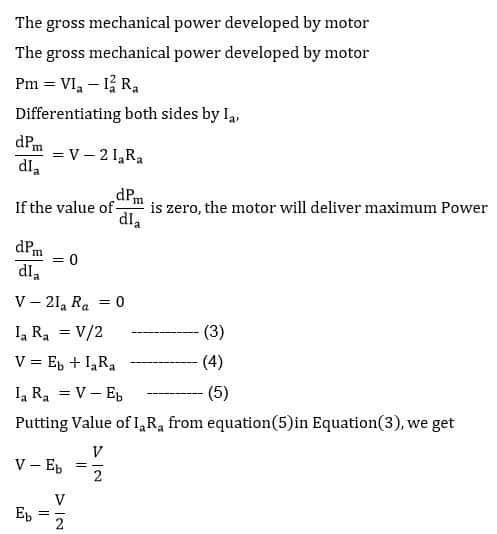# Condition for Maximum Power of DC Motor

The DC motor is most suitable for the loads which demand higher starting torque. When DC voltage is applied to armature the motor is at standstill and no back EMF is induced across the armature, and as a result the motor draws large current from the supply source and delivers higher starting torque as torque is proportional to armature current.The voltage applied to armature overcomes the back EMF and armature drop in the armature winding. As per Kirchhoff’s voltage law all the voltages around a closed loop in a circuit is equal to zero.The equation (1) is called voltage equation of DC motor.

Power Equation of DC Motor

The power developed by motor can be determined by multiplying the voltage applied (V) to armature current(Ia).By Multiplying both sides of equation(1) by Ia, we get power equation of DC Motor.Thus the efficiency of DC motor depends on the back EMF (Eb) and the motor has higher efficiency if back emf is more.

Condition for Maximum PowerThus the motor will deliver maximum power when the back EMF is equal to half of the applied armature voltage.

However, this condition can not be realized. If the back EMF is half of the applied armature voltage, the motor will draw more current than its rated current and efficiency of the motor will be below 50 percent.

Related Posts:

1.